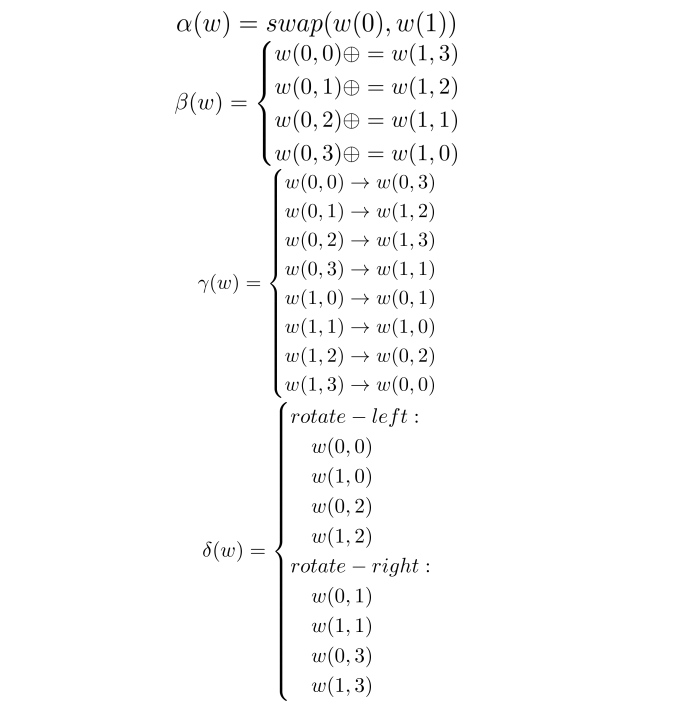# RITSEC CTF 2018: Cictrohash

## November 18, 2018

I didn’t plan to play this CTF but when I saw this challenge I was a bit hooked. So I ended up competing in it.

See the attached PDF for an amazing new Cryptographic Hash Function called CictroHash. For this challenge you must implement the described Hash Function and then find a collision of two strings. Once a collision is found send both strings to fun.ritsec.club:8003 as a HTTP POST request like below:

``````    curl -X POST http://fun.ritsec.club:8003/checkCollision \
--data '{"str1": "", "str2": ""}'
If the strings are a valid collision then the flag will be returned.
``````

The attached PDF itself resembled an academic description of a sponge hash with very detailed implementation information.

In order to solve this I decided to try and implement the hash function and then find a collision locally. The important parts of the descriptions were:

1. The starting state of r and c (denoted S) is shown below.

`S = {31, 56, 156, 167, 38, 240, 174, 248}`

2. Cictrohash `r` is a 4 byte hash, `c` is also 4 bytes.

3. The hash digest itself is z0 in the diagram and is the lower 4 bytes only.

4. The hash uses 50 rounds of a transformation function `f` which operates on a 2 x 4 matrix `w = [[S0,S1,S2,S3],[S4,S5,S6,S7]]`

5. `f` consists of four other functions named alpha, beta, gamma, and delta. They have the following constructions:1. The rotate left and right operations are by 1 bit.

With all of this the actual code is relatively simple. I wrote in Python:

``````#!/usr/bin/python

# Start values of r  and  c
r = [31,56,156,167]
c = [38,240,174,248]

rounds = 50

# the transformations
# alpha(w) = swap(w(0),w(1))
def alpha(w):
return [w,w]

# beta(w) = xor ops
def beta(w, debug=False):
w = w ^ w
w = w ^ w
w = w ^ w
w = w ^ w
return w

# gamma(w) = map ops
def gamma(w, debug=False):
new_w = [list(w),list(w)]
new_w = w
new_w = w
new_w = w
new_w = w
new_w = w
new_w = w
new_w = w
new_w = w
return new_w

# delta(w) = rol/ror ops
def delta(w):
w = rol(w)
w = rol(w)
w = rol(w)
w = rol(w)
w = ror(w)
w = ror(w)
w = ror(w)
w = ror(w)
return w

def round(S):
w = [S[0:4],S[4:8]]
for i in range(rounds):
w = delta(gamma(beta(alpha(w))))

return w+w

# helper functions for the bitwise rotations
def rol(val, bits=1, bit_size=8):
return (val << bits % bit_size) & (2 ** bit_size - 1) | \
((val & (2 ** bit_size - 1)) >> (bit_size - (bits % bit_size)))

def ror(val, bits=1, bit_size=8):
return ((val & (2 ** bit_size - 1)) >> bits % bit_size) | \
(val << (bit_size - (bits % bit_size)) & (2 ** bit_size - 1))

# xor block takes a 4 byte string P and returns P ^ r
def xorblock(P, S):
if len(P) < 4:
while len(P) < 4:
P += '\x00'

for i in range(len(P)):
S[i] = ord(P[i]) ^ S[i]

return S

def cictrohash(input):
# S state array seeded with starting values
S = r + c

# Absorbing
for i in range(0,len(input),4):
try:
# try send a 4 byte block
S = xorblock(input[i:i+4], S)
except IndexError:
# send as many blocks as we have then
S = xorblock(input[i:], S)

S = round(S)

# Squeezing
z = "0x%.2x%.2x%.2x%.2x" % (S, S, S, S)

return z

print cictrohash("HELLOWORLD")

``````

When we run it we get a confirmation that our hash matches the documented example for `HELLOWORLD`:

``````\$ ./cictrohash.py
0xb5a79bee
``````

Great how do we get a collision? The first thing I thought of was to just try flipping individual bits in the input to get a hash collision. So I add the following additional code to the Python:

``````# Try single bit flips to find a collision
import libnum

startstring = "HELLOWORLDHELLOWORLDHELLOWORLDHELLOWORLDHELLOWORLDHELLOWORLDHELLOWORLDHELLOWORLDHELLOWORLDHELLOWORLDHELLOWORLDHELLOWORLDHELLOWORLDHELLOWORLD"

# toggle the n'th bit of an integer.
def toggle_bit(i,offset):

target = cictrohash(startstring)

print '[*] Target:', target
ss_num = libnum.s2n(startstring)

for i in range(ss_num.bit_length()):
a = toggle_bit(ss_num, i)
h = cictrohash(libnum.n2s(a))

if h == target and libnum.n2s(a) != startstring:
print "[+]",startstring
print "[+]",libnum.n2s(a),"works"
break

``````
``````\$ ./chbitflip.py
[*] Target: 0xcb7e588c
[+] HELLOWORLDHELLOWORLDHELLOWORLDHELLOWORLDHELLOWORLDHELLOWORLDHELLOWORLDHELLOWORLDHELLOWORLDHELLOWORLDHELLOWORLDHELLOWORLDHELLOWORLDHELLOWORLD
[+] HELLOWORLDHELLOWORLDHELLOWORLDHELLOWORLDHELLOWORLDHELLOWORLDHELLOWORLDHELLOWORLDHELLOWORLDHELLOWORLDHELLOWORLDHELLOWORLEHELLOWORLDHELLOWORLD works

``````

I submit the two strings to the server and get the flag! Woot!

### DUCTF 2023 Writeups

This weekend I played DownUnderCTF 2023. The fourth instalment of the now huge DownunderCTF. This round they provided a new category of b...… Continue reading

#### LACTF 2023 Writeups

Published on February 12, 2023

#### BlueHens CTF 2022 PWN Writeups

Published on October 30, 2022